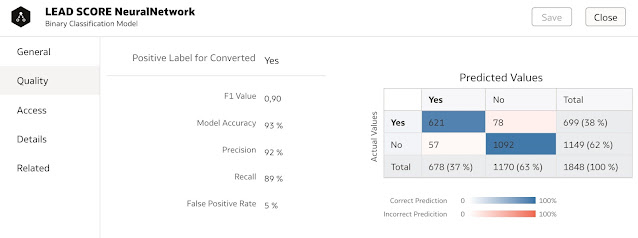Assessing the quality of predictive models in Oracle Analytics using Lift & Gain

Anyone who has done some machine learning modelling in Oracle Analytics, might have been missing a bit more of quality metrics to better assess the quality of trained models.

By default any generated classification model would enable users to evaluate trained models by reviewing Quality metrics such as Precision, Recall, Accuracy, F1 and False Positive Rate.

When presenting Machine Learning support within Oracle Analytics, the question about calculating Gain and Lift are often asked. Until now, Oracle Analytics didn’t support this functionality and this has changed in the recent release of Oracle Analytics 6.3.

Lift and Gain Analysis

In order to measure how the prediction model is better then not using a prediction model at all, Lift and Gain Analysis is used. Using other words, with Lift and Gain analysis we can find out what is the benefit of applying predictions based on a prediction model to the business.

In Oracle Analytics, Lift and Gain Analysis is performed by visualizing model statistics in a Data Visualization Workbook.

This blog contains several examples of how machine learning models can be trained and used for predictions. For example, quite some time ago I presented a “classic” example of classification done in Oracle Analytics, churn prediction in the blog series Telco Churn Prediction in Oracle Analytics Revisited.

One of the key steps in the Machine Learning process is of course model training and evaluation. Oracle Analytics Data Flows functionality is the place where all magic happens. From Oracle Analytics 6.3 on, the Apply Model step includes also the functionality to create dataset for Lift and Gain information.

Before we explore a little bit more in details, let me only mention, that Oracle Autonomous Database is a prerequisite.

Training a new machine learning model

In the following example, I am using the Lead Scoring dataset from Kaggle. The data set has been cleaned and prepared for the machine learning model building using Data Flows:

Then Data Flows was used again for model creation:

In the example above, Neural Networks algorithm was used, but as you probably know, Oracle Analytics supports other classification algorithms as well, algorithms such as Naive Bayes, SVM, CART, Logistic Regression and Random Forest

Confusion Matrix and Quality Metrics reveal that “out-of-the-box” Neural Network model performs quite well (for example, several data scientists on Kaggle reported 94% as the best result for accuracy by using Python machine learning).Just for comparison, Random Forest model on the other hand was not performing that well. Actually it did relatively poor comparing to the Neural Networks:

This approach gives us relatively good picture about which model would perform better. In our example, it is the first model. However sometimes this picture is not so obvious. That is why, lift and gain analysis could be very helpful.

Calculating Lift & Gain

Apply Model step in a data flow is one way of deploying trained models and making predictions on a new set of data. In this step additional parameters have now been added to calculate Lift and Gain

And then in the last step, the standard Save Data step is applied.

When data flow is run, data set LEAD_SCORING_NN with predictions is created. Besides this data set, we can see that there is another dataset created LEAD_SCORING_NN_LIFT.

The “lift” data set contains the following information (more details can be found here):

• PopulationPercentile - The dataset population split into 100 equal groups.
• CumulativeGain - The ratio of the cumulative number of positive targets up to that percentile, to the total number of positive targets. The closer the cumulative gains line is to the top-left corner of the chart, the greater the gain; the higher the proportion of the responders that are reached for the lower proportion of customers contacted.
• GainChartBaseline - The overall response rate : the line represents the percentage of positive records we expect to get if we selected records randomly. For example, in a marketing campaign, if we contact X% of the customers randomly, we will receive X% of the total positive response.
• LiftChartBaseline - Value of 1 and used as a baseline for lift comparison.
• LiftValue - The cumulative lift for a percentile. Lift is the ratio of the cumulative positive records density for the selected data, to the positive density over all the test data.
• IdealModelLine - The ratio of the cumulative number of positive targets to the total number of positive targets.
• OptimalGain - This indicates the optimum number of customers to contact. The cumulative gain curve will flatten beyond this point.

As you can see, data is prepared by percentiles, which can be easily visualised:

Cumulative Gain is displayed on the left chart. The lines represent the following:

• The base line is the line which represents the situation when no prediction model is applied.
• The ideal line which represents the best possible outcome.
• The cumulative gain which represents how well the model is performing. We can see that in 39th percentile the model reaches its optimum, the optimal gain.

Similarly, we see the results in the lift chart, which is on the right side. We can see that lift starts dropping dramatically after 39th percentile. Using other words, we should address only those leads which fall between 1st and 39th percentile.

If we now plotted also results of the Random Forest model, we could compare the two models among themselves.

We can conclude that there is no doubt that Neural Network model is performing better over Random Forest. We can also observe, that the first model achieves its optimum at 39th percentile, whereas the second at 49th percentile.

I think this “little” feature would help business analysts a lot when they are deploying their own machine learning models in Oracle Analytics.